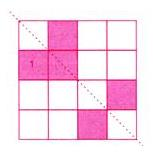Courses

# Test : Symmetry - 2

## 10 Questions MCQ Test Mathematics for Class 5: NCERT | Test : Symmetry - 2

Description
This mock test of Test : Symmetry - 2 for Class 5 helps you for every Class 5 entrance exam. This contains 10 Multiple Choice Questions for Class 5 Test : Symmetry - 2 (mcq) to study with solutions a complete question bank. The solved questions answers in this Test : Symmetry - 2 quiz give you a good mix of easy questions and tough questions. Class 5 students definitely take this Test : Symmetry - 2 exercise for a better result in the exam. You can find other Test : Symmetry - 2 extra questions, long questions & short questions for Class 5 on EduRev as well by searching above.
QUESTION: 1

### How many of the following letters have atleast one line of symmetry?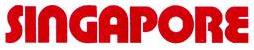Solution: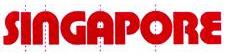QUESTION: 2

### Which square must be shaded so that the figure has a line of symmetry?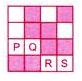Solution: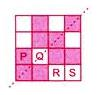QUESTION: 3

### Which square must be shaded so that the figure has a line of symmetry?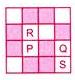Solution: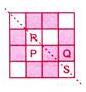QUESTION: 4

Which of the following dotted lines is a line of symmetry?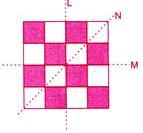Solution:
QUESTION: 5

Which of the squares must be shaded so that the dotted line AB becomes the line of symmetry?
​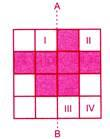Solution: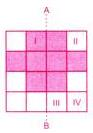QUESTION: 6

What is the smallest number of squares that must be added so that the line PQ becomes a line of symmetry?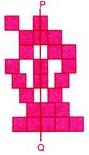Solution: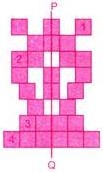QUESTION: 7

​What is the smallest number of squares that must be shaded so that the figure has a line of symmetry?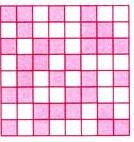Solution: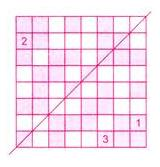QUESTION: 8

What is the smallest number of squares that must be added so that the line AB becomes a line of symmetry?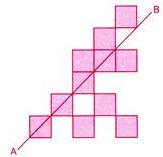Solution: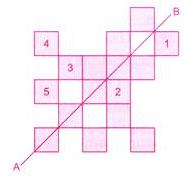QUESTION: 9

How many Lines of symmetry does the figure have?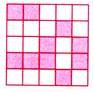Solution:
QUESTION: 10

The minimum number of squares that must be shaded, so that the figure has a line of symmetry is ________.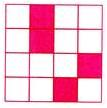Solution: